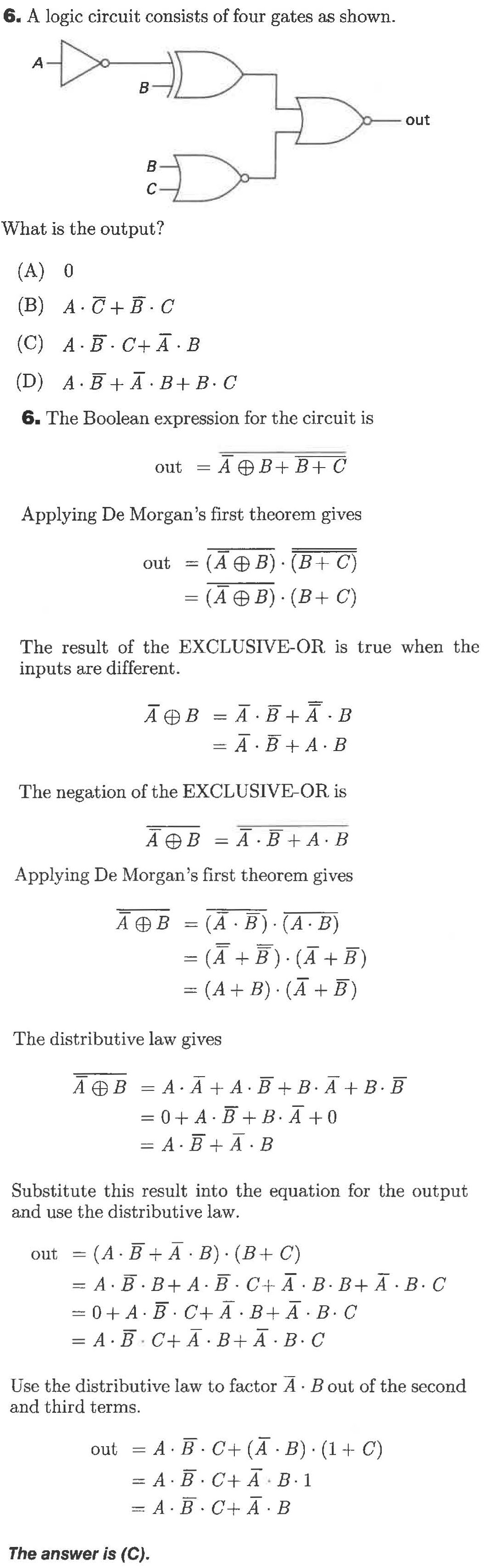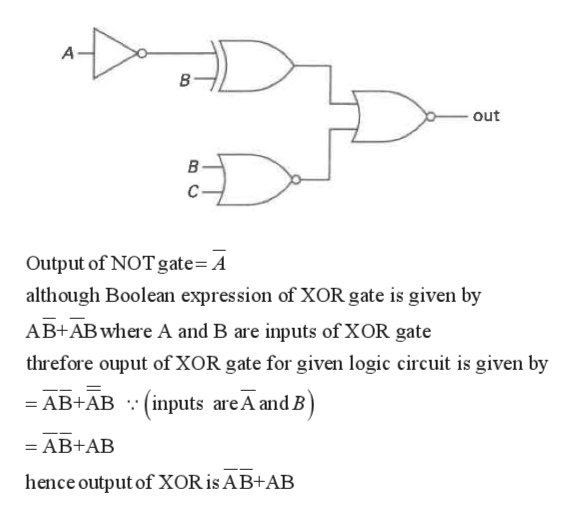# 6. A logic circuit consists of four gates as shownAВoutВСWhat is the output?(A) 0(B) A CB. C(C) A B C+A B(D) A.BA B+B. C6. The Boolean expression for the circuit isA BB+CoutApplying De Morgan's first theorem gives(А Ф В): (В+ C)out(А Ө В). (В+ С)The result of the EXCLUSIVE-OR is true when theinputs are differentA BA BАӨВ= A BA BThe negation of the EXCLUSIVE-OR isA B = A B+ABApplying De Morgan's first theorem gives(A B) (A B)(А + B) (A + В)A B- (A B) (AB)The distributive law givesAAA.B B. AB.BАФВ= 0 + A BB. A 0= A. BA. BSubstitute this result into the equation for the outputand use the distributive law= (A B A B) (B C)= A B.B+ A B C+ A B. BA. B. C= 0 + A BC+ A B+ A B. C= A. B C+ A B+A B. CoutUse the distributive law to factor A Bout of the secondand third terms.= A B. C+ (A B) (1 C)outA BC+ A B.1=A B. C+ A BThe answer is (C).

Question
1 views

Hi,

the attached image shows a question and its answer but still, I couldn't understand it please simply explain ithelp_outlineImage Transcriptionclose6. A logic circuit consists of four gates as shown A В out В С What is the output? (A) 0 (B) A CB. C (C) A B C+A B (D) A.BA B+B. C 6. The Boolean expression for the circuit is A BB+C out Applying De Morgan's first theorem gives (А Ф В): (В+ C) out (А Ө В). (В+ С) The result of the EXCLUSIVE-OR is true when the inputs are different A BA B АӨВ = A BA B The negation of the EXCLUSIVE-OR is A B = A B+AB Applying De Morgan's first theorem gives (A B) (A B) (А + B) (A + В) A B - (A B) (AB) The distributive law gives AAA.B B. AB.B АФВ = 0 + A BB. A 0 = A. BA. B Substitute this result into the equation for the output and use the distributive law = (A B A B) (B C) = A B.B+ A B C+ A B. BA. B. C = 0 + A BC+ A B+ A B. C = A. B C+ A B+A B. C out Use the distributive law to factor A Bout of the second and third terms. = A B. C+ (A B) (1 C) out A BC+ A B.1 =A B. C+ A B The answer is (C). fullscreen
check_circle

Step 1

Although, given logic circuit consist of four gates

First one is NOT gate,second is XOR gate, third and fourth is NOR gate.

Now calculating output of logic gates one by onehelp_outlineImage TranscriptioncloseA В out В Output of NOT gate= A although Boolean expression of XOR gate is given by AB+AB where A and B are inputs of XOR gate threfore ouput of XOR gate for given logic circuit is given by =AB+AB (inputs areA and B = AB+AB hence output of XOR is AB+AB fullscreen
Step 2

Similarly output of NOR gate which inputs are B and C is given by

Step 3

Hence outputs of XOR and first NOR gate is the input of second NOR g...

### Want to see the full answer?

See Solution

#### Want to see this answer and more?

Solutions are written by subject experts who are available 24/7. Questions are typically answered within 1 hour.*

See Solution
*Response times may vary by subject and question.
Tagged in

### Electrical Engineering